CBSE Class 10 Sample Paper for 2020 Boards - Maths Standard

Class 10
Solutions of Sample Papers for Class 10 Boards

## Construct a pair of tangents to a circle of radius 3 cm which are inclined to each other at an angle of 60°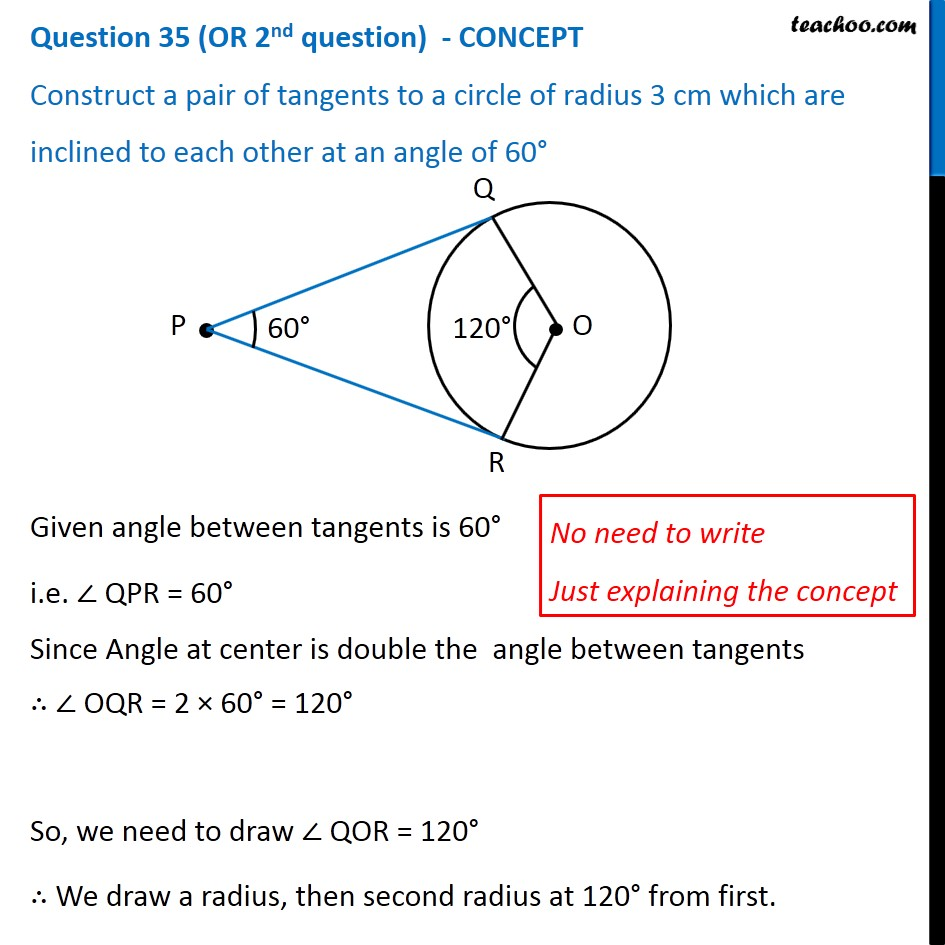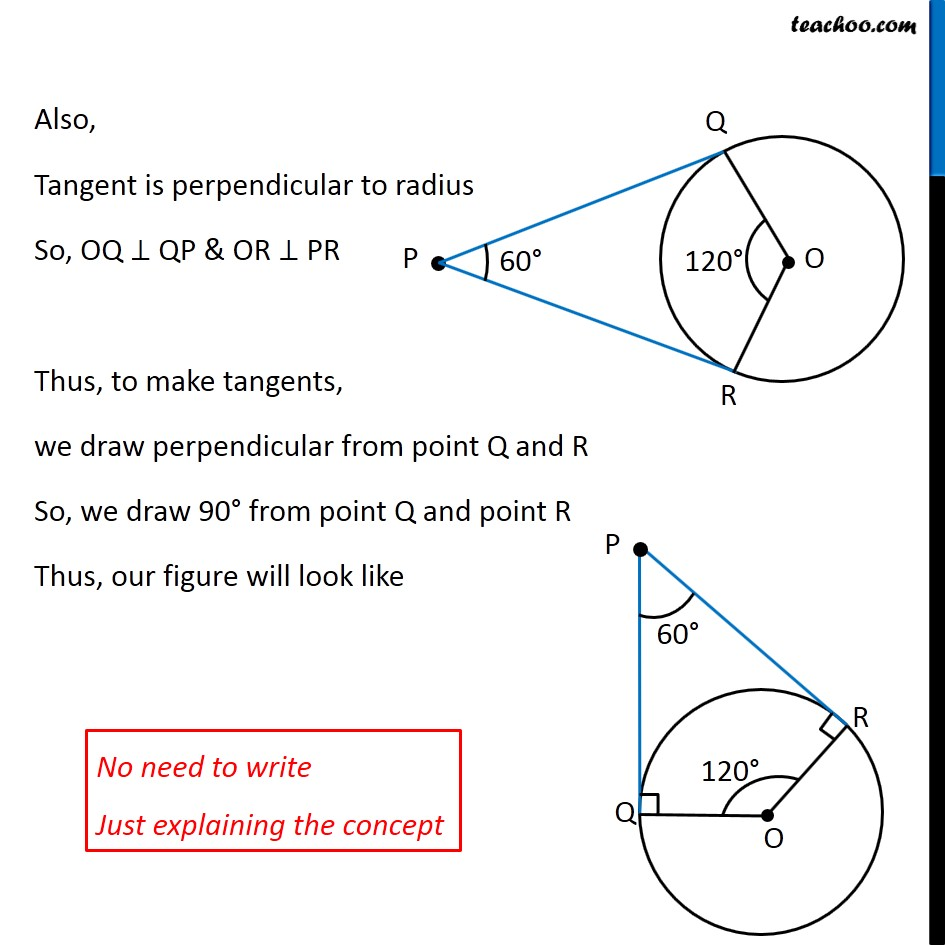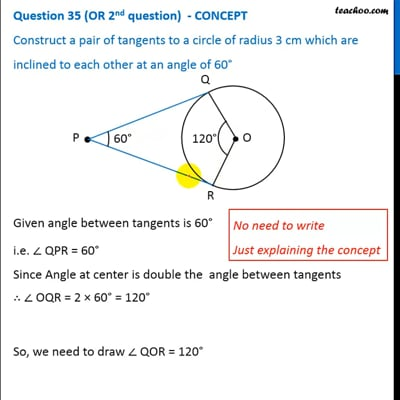This video is only available for Teachoo black users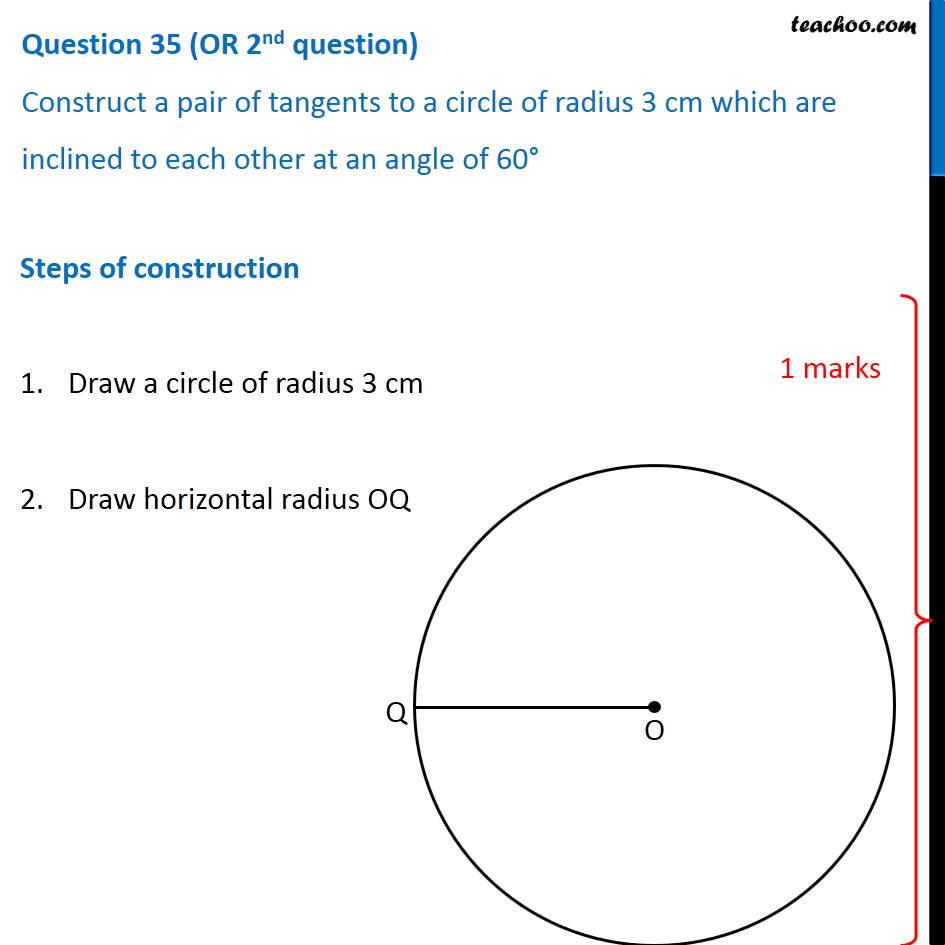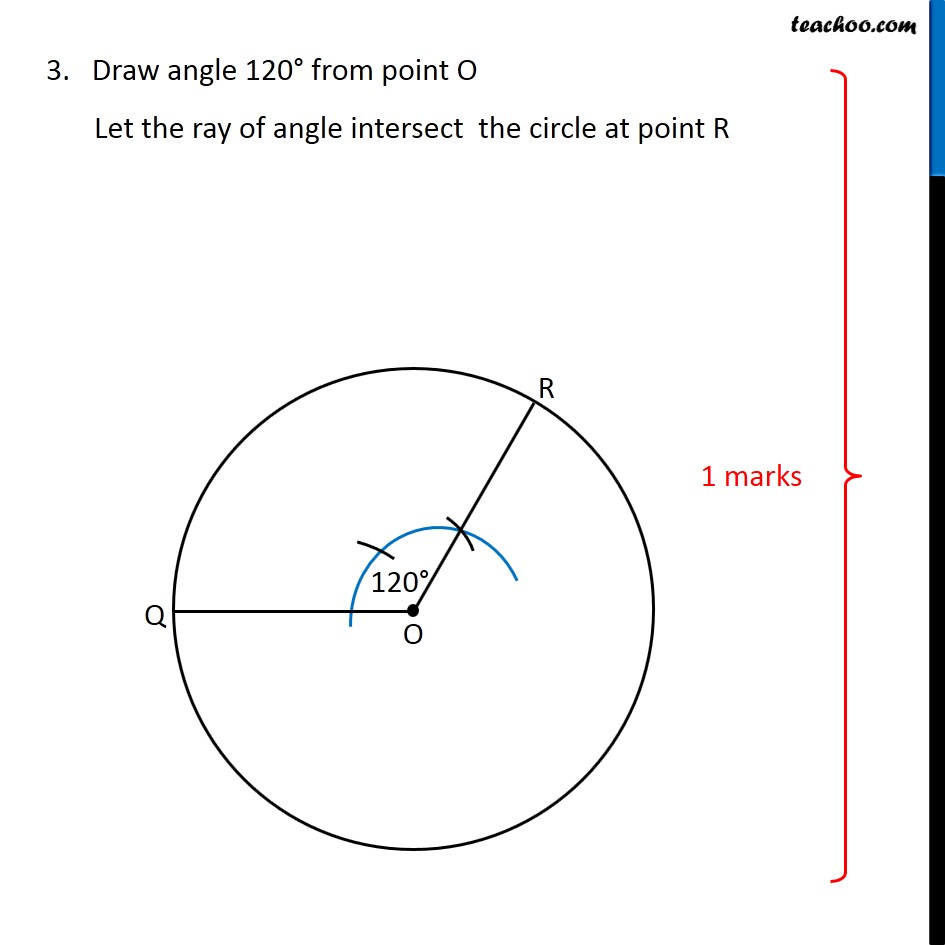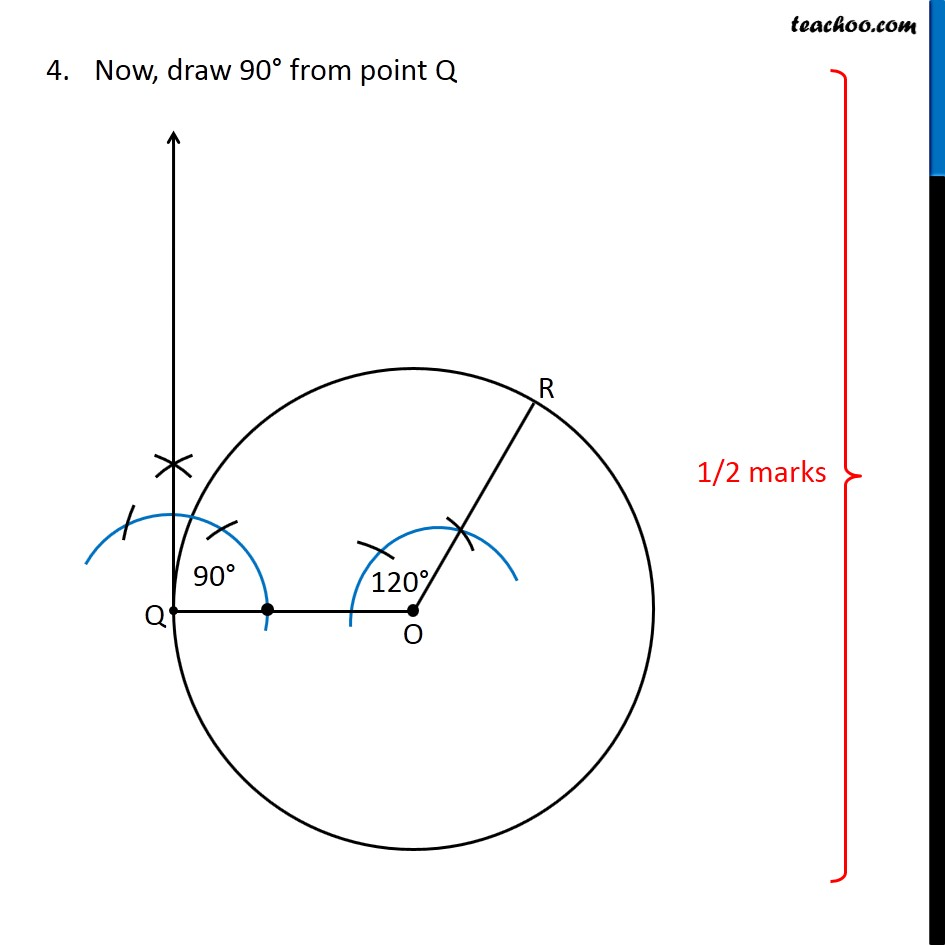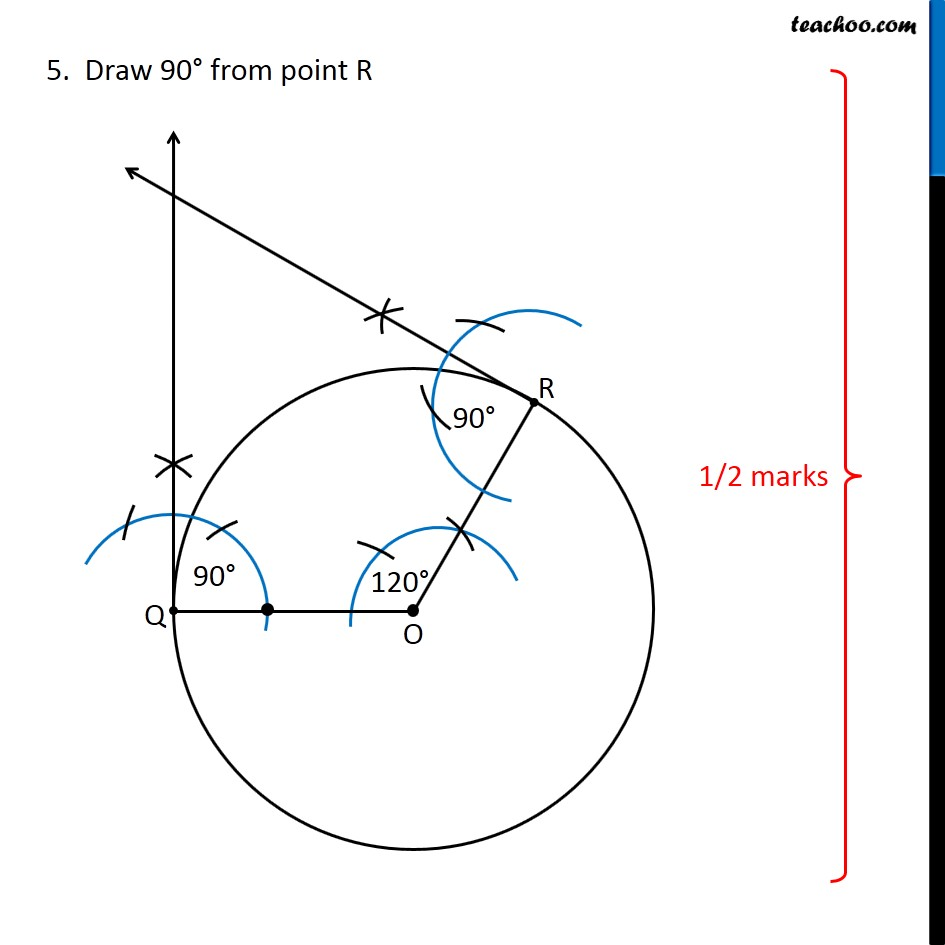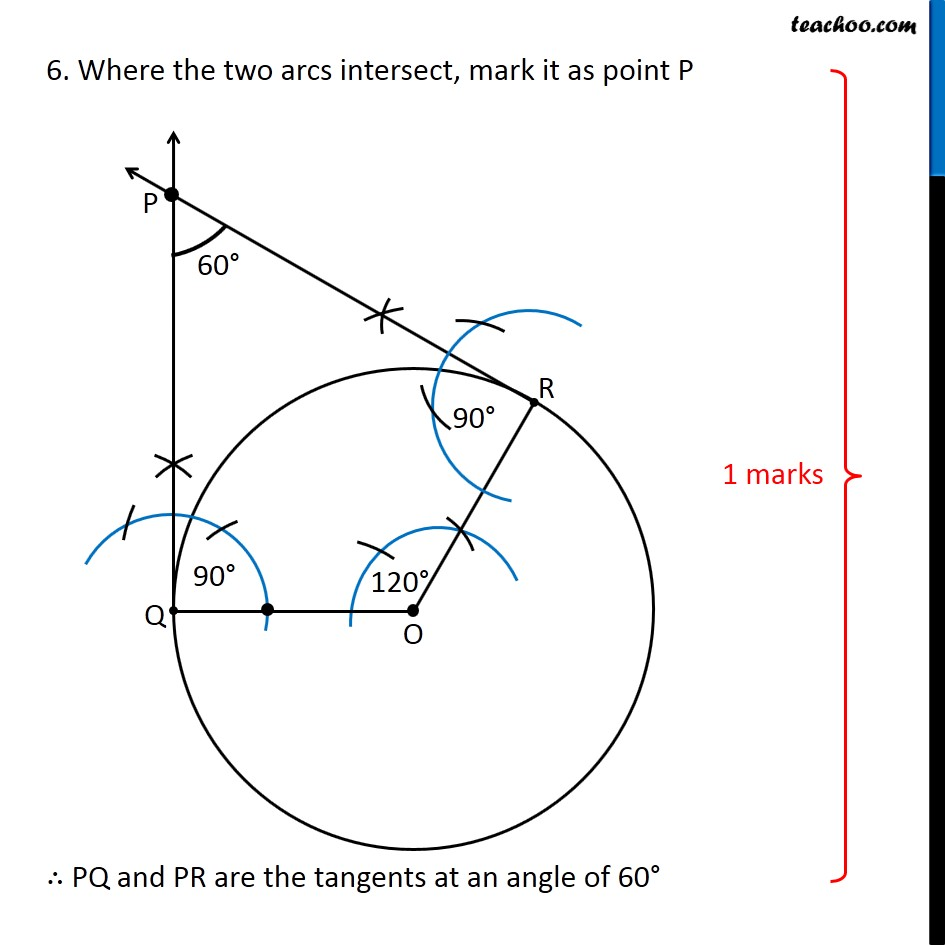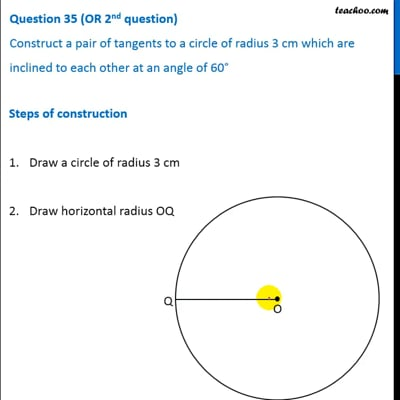This video is only available for Teachoo black users

Note : This is similar to Ex 11.2, 4 of NCERT – Chapter 11 Class 10

Learn in your speed, with individual attention - Teachoo Maths 1-on-1 Class

### Transcript

Question 35 (OR 2nd question) - CONCEPT Construct a pair of tangents to a circle of radius 3 cm which are inclined to each other at an angle of 60° Given angle between tangents is 60° i.e. ∠ QPR = 60° Since Angle at center is double the angle between tangents ∴ ∠ OQR = 2 × 60° = 120° So, we need to draw ∠ QOR = 120° ∴ We draw a radius, then second radius at 120° from first. Also, Tangent is perpendicular to radius So, OQ ⊥ QP & OR ⊥ PR Thus, to make tangents, we draw perpendicular from point Q and R So, we draw 90° from point Q and point R Thus, our figure will look like Question 35 (OR 2nd question) Construct a pair of tangents to a circle of radius 3 cm which are inclined to each other at an angle of 60° Steps of construction Draw a circle of radius 3 cm Draw horizontal radius OQ 3. Draw angle 120° from point O Let the ray of angle intersect the circle at point R Now, draw 90° from point Q 5. Draw 90° from point R 6. Where the two arcs intersect, mark it as point P ∴ PQ and PR are the tangents at an angle of 60°